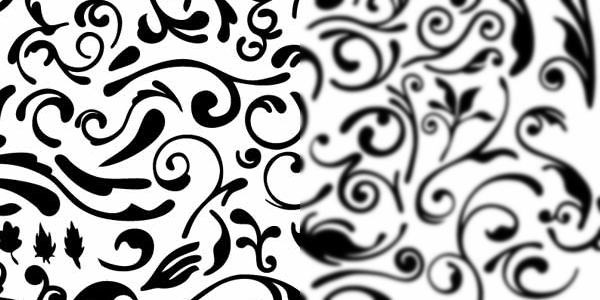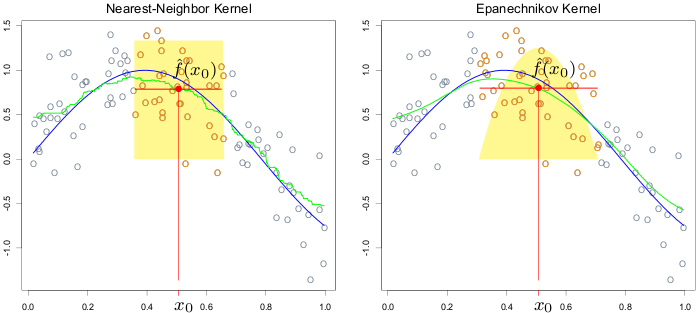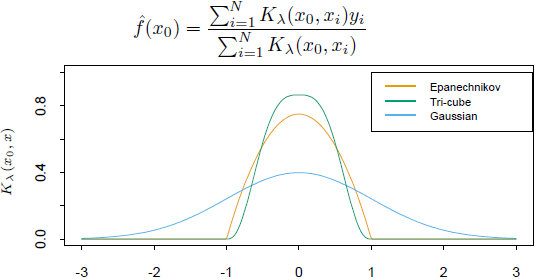## 模糊和平滑

2010 年 02 月 24 日 | 分类于 学习中#生成“框”中各个元素的权重，grid是框的边长。
gaussm = function(grid, sigma) {
m = outer(1:grid, 1:grid, function(x, y) dnorm(x - (grid +
1)/2, 0, sigma) * dnorm(y - (grid + 1)/2, 0, sigma))
return(m/sum(m))
}
#加载rimage包。
library(rimage)
#指定框的边长和sigma的取值。
grid = 5
sigma = 3
#生成框。
m = gaussm(grid, sigma)
#读取图片，这段程序中需要灰度的jpg或jpeg图片。
#把图片转成像素矩阵。
picm = pic[, ]
newm = picm
r = (grid - 1)/2
#对像素点逐一进行转换，为了简便起见没有包括图片边缘上的点。
for (i in (r + 1):(ncol(picm) - r)) {
for (j in (r + 1):(nrow(picm) - r)) {
newm[j, i] = sum(picm[(j - r):(j + r), (i - r):(i + r)] *
m)
}
}
#画出原来的图片。
plot(pic)
#新开一个窗口，画出高斯模糊后的图片。
x11()
plot(imagematrix(newm))


blur<-function(x,grid,kernelf,...)
{
if(grid<3) stop("grid should be great than or equal to 3!");
if(grid%%2==0) stop("grid should be odd number!");
v=sapply(1:grid-(grid+1)/2,kernelf,...);
v<-v/sum(v);
r<-(grid-1)/2;
newx<-tmp<-x;
is.grey<-length(dim(x))<3;
for(i in (r+1):(nrow(x)-r))
{
if(is.grey)
{
tmp[i,]<-colSums(x[(i-r):(i+r),]*v);
}else{
for(k in 1:3)
{
tmp[i,,k]<-colSums(x[(i-r):(i+r),,k]*v);
}
}
}
for(j in (r+1):(ncol(x)-r))
{
if(is.grey)
{
newx[,j]<-colSums(t(tmp[,(j-r):(j+r)])*v);
}else{
for(k in 1:3)
{
newx[,j,k]<-colSums(t(tmp[,(j-r):(j+r),k])*v);
}
}
}
return(newx);
}
kernel.gauss<-function(x,sigma) dnorm(x,0,sigma);
blur.gauss<-function(x,grid,sigma) blur(x,grid,kernel.gauss,sigma=sigma);

library(rimage);